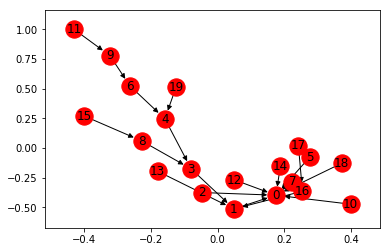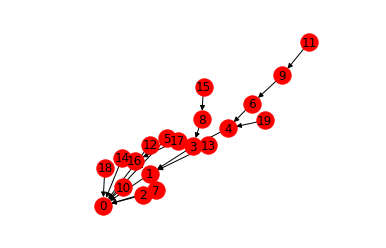# Goulib.graph¶

efficient Euclidian Graphs for NetworkX and related algorithms

In :
from Goulib import notebook
import networkx as nx
from Goulib.graph import *

In :
g=nx.gn_graph(20)
nx.draw_networkx(g)In :
draw_networkx(g, #improved version over nx.draw_networkx
labels=True, # by default they're off
)

Out:In :
geo=nx.geographical_threshold_graph(50,25)
geo # NetworkX Graphs do not render in Notebooks...

Out:
<networkx.classes.graph.Graph at 0x1c7b5b265f8>
In :
geo=GeoGraph(geo)
geo # GeoGraphs do !

C:\Anaconda3\lib\site-packages\networkx\drawing\nx_pylab.py:611: MatplotlibDeprecationWarning: isinstance(..., numbers.Number)
if cb.is_numlike(alpha):

Out:
In :
# it's easy to make GeoGraphs look nice
def edge_color(data): # simply define a function that maps edge data to a color
return plt.get_cmap('Blues')(data['length']/.25)
geo.render(edge_color=edge_color, node_size=50) #this will set geo.render_args ...
geo #... so here we display them :-)

Out:
In :
d=to_drawing(geo) # graphs can also be converted or added to a Goulib.Drawing
from Goulib.geom import Circle
c=Circle((0.5,0.5),0.5)
c.color='red'
d.append(c)

Out:
In :
nodes=points_on_sphere(120)
sphere=GeoGraph(nodes=nodes) #GeoGraphs can also be built from n-dim points only
sphere.render(edge_color=edge_color, node_size=50) #this will set geo.render_args ...
sphere

Out:
In :
delauney_triangulation(sphere,'Qz') #creates a new GeoGraph from Delauney triangulation of a GeoGraph's nodes

Out:
In [ ]: